HOME AUTHOR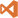MIXEDIT +ARTICLES +TUTORIALS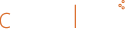# Article - Gamma and Linear Spaces

## Gamma correction for 3D graphics

Gamma correction is a characteristic of virtually all modern digital displays and it has some important implications to the way we work with images. The term gamma is often used in a confusing way as it gets mapped to different concepts, so in this article I will always put a second word with it to provide more context; the word gamma itself comes from the exponent of the encode/decode function used by the non-linear operation known as gamma correction. $y=x^\gamma$

The reason why we have gamma correction in the first place goes back to CRT displays. The technology used by these monitors to light  the pixels introduced a non linear conversion from the energy in input to the energy in ouput. This means that if we send a value of 128 to the monitor it wouldn't appear 50% the maximum brightness of the monitor but it would be sensibly darker. To actually get a linear response so that our 128 looks like a mid gray we need to apply the inverse of the monitor's transformation to the input (intuitively, if 128 is too dark we send in a bigger number to compensate). The transformation function is the non linear operation $y=x^\gamma$ where $x$ is our input value, $y$ is what we see on monitor and $gamma$ is the correction value that depends on the physical technology used in the CRT. Why we are still interested in this then? We don't use CRT monitors anymore! Well, because of a fortunate coincidence by using that operation we also improve the visual quality of the signal as we happen to allocate more information in the areas where the human eye is more sensitive (darker shades).

The non linear operation that the display applies to the signal is called gamma decode or gamma expansion. The operation that we apply to compensate this is called gamma encode or gamma correction. The function used for these operations is always the same $y=x^\gamma$ and the only thing that changes is the gamma parameter; usually for decoding it's set to 2.2, and for encoding it's $\frac{1}{2.2}$.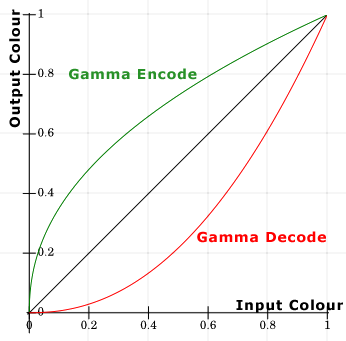Figure: Plot of the two operations with a gamma value of 2.2 and  $\frac{1}{2.2}$

The reason why all this is important for computer graphics is that we work with different elements that expect the signals to be in a certain space. The display expects the signal to be gamma encoded since it will apply a gamma decode to it, and because of this the graphics tools we use do store the images after applying the gamma correction. This means that virtually all the images we have on the hard disk are stored after gamma correction and if we could open them on a linear display we would see them more bright than we would expect.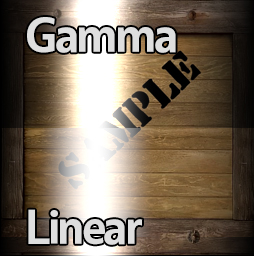Figure: Same asset shaded with and without the gamma correction applied

## First Step

Let's see one problem at the time. The first issue is feeding the monitor with the right input. We know that the monitor is going to apply a gamma expansion to anything we send to it. If we don't encode the signal and send a linear input to the monitor this will get gamma decoded without ever being gamma encoded, producing a different result from what we actually want.This means that if we have a sphere lit by a point light and shaded with a simple lambert equation we would get the following visual output:

$shader\_output=dot(N,L)$

Monitor Decode: $monitor\_output = shader\_output ^ {2.2} = dot(N,L) ^ {2.2}$

Which is not what we want! To actually get the dot product to the monitor without the extra exponent operation we need to apply the gamma encode first, which gives us:

$shader\_output=dot(N,L) ^{ \frac{1}{2.2} }$

Monitor Decode: $monitor\_output = (shader\_output^\frac{1}{2.2}) ^ {2.2} = dot(N,L)$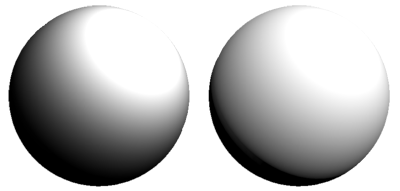Figure: Left lambert shading without gamma encoding, Right with gamma encoding

Now the monitor is showing the desired output. To get the right result from the shader we have to pay for an extra pow function, which is not ideal, but fortunately there is another way to obtain the same results by notifying the hardware of what is going on. In DirectX 11 we can flag the back buffer with a format that ends with _SRGB to tell the driver to do the encode for us when we write to it.

## Second Step

So now we know how to provide the right input to the monitor, but there is another problem that needs addressing: texture reads. As we said the graphics tools we use tend to save their data after gamma encoding it. This is quite imprecise though as what really happens is that depending on your tool's settings you will see the image with or without the encoding applied. If no encoding is applied any colours you see will be darker so to compensate you will end up picking brighter colours than normal, which means that the image saved on disk will be brighter. Camera devices do a similar thing by gamma encoding the picture before saving it to the memory card. Now imagine what would happen if you were to read a texture as it comes from the disk and then render it to the screen. Let's see it in pseudo-code with the gamma correction applied for the monitor:

$shader\_output = tex2D(diffuse\_sampler, UV) = original\_pixel ^ \frac{1}{2.2}$

$monitor\_output = (shader\_output^\frac{1}{2.2}) ^ {2.2} = ((original\_pixel ^ \frac{1}{2.2})^\frac{1}{2.2}) ^ {2.2} = original\_pixel ^ \frac{1}{2.2}$Original pixel is the value we painted into the image, while $original\_pixel ^ \frac{1}{2.2}$ is what the software has saved to the disk and therefore the value we read back. As you can see the output is wrong as it is the original pixel as saved from the software, therefore with the gamma encode applied. This means that every texture we read that doesn't contain generated data (so normal maps, height maps and so on are usually not affected since probably they are generated from some software and saved without the gamma correction) needs to be gamma decoded. In pseudo code:

$shader\_output = pow( tex2D(diffuse\_sampler, UV), 2.2) = (original\_pixel ^ \frac{1}{2.2}) ^ {2.2} = original\_pixel$Also in this case the hardware allows us to read the texture while gamma decoding it. In DirectX 11 we can flag the texture we read from with a format that ends with _SRGB to tell the driver to do the decode for us when we read from it.

## Results

To try and clarify what happens if you get the encode and decode sequence wrong I have put together a simple test that combines all the possible combinations you can get with a single texture and the backbuffer in Direct X 11.
The black and white stripes pattern can be used to figure out what gamma you are seeing. Assuming that your browser hasn't resized the image, if you step back enough the stripes will became undistinguishable and you will see just a solid colour which supposedly is your mid gray (you may want to open the image in a tool that doesn't resize it to better appreciate the effect). Because gamma decoding pure black gives pure black, and decoding pure white gives pure white the colour we see is effectively your actual mid gray.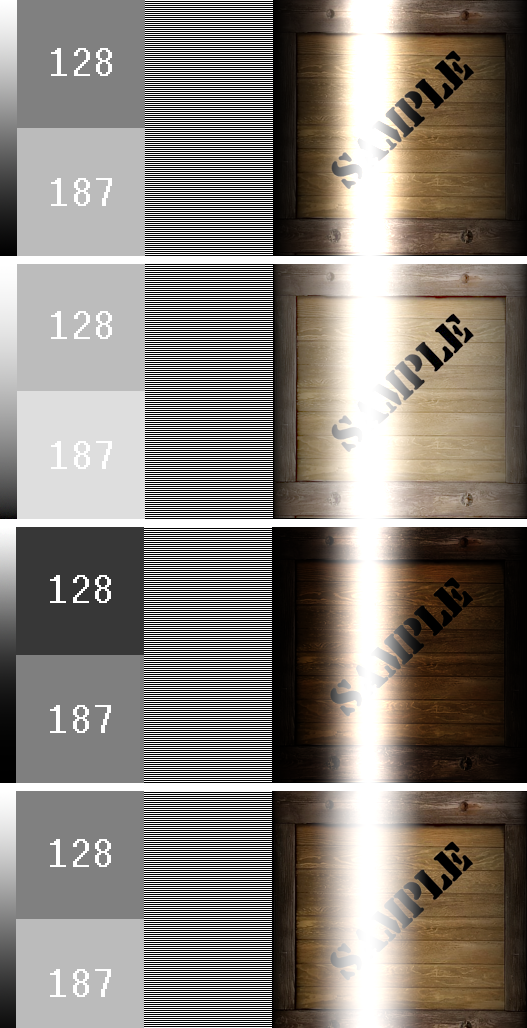The first case is the classic case that we have been running until all this gamma correction sequence has been properly understood, and as you can see it's mostly fine. What it's worth to point out is that the diffuse falloff has quite a lot of black in it and that the specular is "burned" and is not pure white (even if we are adding the same value to all channels!). This is due to the gamma exponent applied at the end by the monitor to our math. The left bit where no math is applied shows correctly. It's important to notice how the striped pattern matches with 187 rather than 128; this is because 187, once sent to the monitor, gets gamma decoded, which translates to $0.73333^{2.2} = ~0.5$ (0.7333 is 187 normalized to 255).

The third case looks darker, and this is because we decode more times than we encode. When we read the texture data we correctly decode it, but then when we send it to the monitor we don't gamma encode it as we should. The monitor is not aware of this and apply its gamma decode anyway, which means we gamma decode a signal that was not encoded. Notice how in this case 187 is not mid gray anymore because the value erroneusly sent to the monitor is 0.5, which is then converted to 0.25 .

The second case is very similar to the third one but it looks bright rather than dark, and this is because we encode more times than we decode.

Finally, the fourth case is doing what we want, it's encoding and decoding the right amount of times and the math in the shader is correctly converted where needed while using the texture data in the correct space.